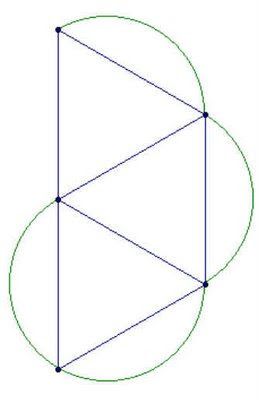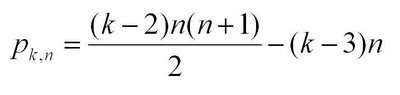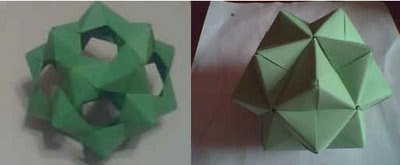## Thursday, August 14, 2008

### Cut-and-Glue Polyhedral ModelsBuilding polyhedral models is a nice way to explore a lot of significant mathematics. The above models were made by printing patterns onto card-stock, cutting them out, and gluing them together. For these models, only triangular faces were used. These can give you a wide variety of cumulated (or augmented) polyhedra. The triangular faces are circumscribed to provide tabs that you glue together. You can fold and glue the tabs so that they are inside the models, but it is easier to leave them out, and they look nice this way (I think).

1. Decide on a model that you would like to make, and figure out how many faces you will need.
2. Copy and paste the images below into a document or presentation slide (PowerPoint works well) for printing. Choose the right ones for your model, and fit as many as you can on a single sheet.
3. Print out onto card-stock. Most desk ink-jet printers can take card-stock instead of printer-paper.
4. Cut out the units, fold the tabs, and assemble and glue.

Throughout this process, it helps if you have pictures of the polyhedra that you want to construct. Poly is a nice software package for browsing through families of polyhedra.

I've found that it works well to bend the tabs using a ruler, that glue-sticks provide the best gluing, and that it helps to hold the model together with binder-clips while assembling.## Monday, August 11, 2008

### Polygonal Number FormulasA few of the posts on this blog have dealt with the polygonal numbers. Polygonal numbers are a mainstay of recreational and school mathematics, providing a nice bridge between numbers and shapes. The diagrams above (created using the instructions in an earlier post) show some of the hexagonal numbers.

This post is intended to provide some (limited) background information on two-dimensional polygonal numbers. A previous post suggested an approach for generating a formula for higher-dimensional polygonal numbers. Future posts will elaborate on these numbers some more.

Some examples of two-dimensional polygonal numbers are:

the triangular numbers: 1, 3, 6, 10, 15, ...
the square numbers: 1, 4, 9, 16, 25, ...
the pentagonal numbers: 1, 5, 12, 22, 35,...
the hexagonal numbers: 1, 6, 15, 28, 45, ...

Comparing the listing for the hexagonal numbers with the diagrams above, you can see how the sequences are built diagrammatically. In general, beginning with a single dot, k-sided polygons are built by adding layers (called gnomons) consisting of k-2 segments, with each segment of the gnomon having one more dot than the segments of the previous layer. In this way, the nth gnomon consists of segments each n dots long, but with k-3 dots shared by adjoining segments (the corners).

The description above can lead you to a recursive formula for k-polygonals, writing p_k,n for the nth k-polygonal number:Unwinding the recursion gives you a summation formula for k-polygonals:Knowing a little about sums gives you the direct formula for k-polygonals:Coming a little out of left-field is this combinatorial formula for k-polygonals:This last formula expresses two ideas: that the triangular numbers correspond to the r=0 column of Pascal's triangle, and that every polygonal number can be "triangulated":The combinatorial formula for p_kn can be generalized to higher-dimensional polygonal numbers (pyrimidal numbers, etc.). See this previous post for some discussion on this.

The recreation here lies in showing that the various formulas for p_k,n are really the same, and then exploring the relationships between the different k-polygonals. A great resource is J.H. Conway and R.K. Guy's The Book of Numbers.

## Wednesday, August 6, 2008

### Sonobe Phizz DualitySonobe and phizz modular origami units are assembled into polyhedral models in similar ways, but the models that they produce are "dual" to each other (vertices in one correspond to faces in the other). Assembling models out of sonobe and phizz units with this duality in mind provides a nice way of exploring duality and the relationships between edges, faces, and vertices.

The sonobe and phizz modules are both examples of edge modules that come together in groups of 3. In both cases, when the units come together in their groups of 3 they meet in a small triangular pyramid. These pyramids in turn come together in clusters of 3 to 6. The essential difference between the phizz and sonobe modules is in how these clusters form. In the phizz, there is a gap between the groups, so the resulting cluster seems to form the edges of a polygon. In the sonobe, the gap between the groups is small, so the cluster seems to form around a point. Consequently, in the phizz, the center of the cluster takes on the role of a polygonal face, while in the sonobe it is takes on the role of a vertex. Meanwhile, the pyramids formed by the groups of three units become raised vertices in the phizz unit, while in the sonobe they become cumulated faces of the resulting polyhedron.

We naturally interpret what phizz modules generate as polyhedral skeletons, while we see the shapes generated by sonobe as cumulated (or augmented) polyhedra. The fact that this different interpretation is based on the size of the gap that forms in the center of the module clusters suggests that seeing an origami model as a particular polyhedron or its dual is, to some extent, a matter of perception and interpretation.

In phizz models, the fact that the modules come together in groups of 3 dictates that the finished models have vertices of degree (or valence) 3, while in the sonobe case, the 3 units come together to make triangular faces. Dual models have the same number of edges, which corresponds to the number of modules required to build the model.

Some of the models that you can build are summarized below. The picture at the top of the post shows the 30-unit phizz dodecahedron and its dual, the 30-unit sonobe icosahedron.